# Catch up on the latest AI articles# Test Accuracy Can Be Inferred From GAN Samples!

3 main points
✔️ Attempt to predict test accuracy using synthetic datasets with GANs
✔️ Demonstrated results that outperform various existing methods
✔️ Identified interesting properties of GANs, such as the fact that their generative distribution is closer to the test set than to the training set

written by Yi ZhangArushi GuptaNikunj SaunshiSanjeev Arora
(Submitted on 28 Nov 2021 (v1), last revised 17 Mar 2022 (this version, v2))

Subjects: Machine Learning (cs.LG)code：The images used in this article are from the paper, the introductory slides, or were created based on them.

## first of all

Predicting test errors from training datasets and networks alone is a significant challenge in deep learning.

In the paper presented in this article, we tackled this problem by using synthetic data generated by a Generative Adversarial Network (GAN) trained on the same training dataset.

The results show that, contrary to the well-known limitations of GANs (e.g., mode collapse), attempts to predict test errors using GANs have been successful.

## Test Performance Prediction with GAN Samples

In the paper, an attempt is made to predict test performance with synthetic data generated by GAN. Here, we denote the training set, the test set, and the synthetic data set generated by GAN as $S_{train}, S_{test}, S_{syn}$, respectively.

Given a classifier $f$ trained on the training set $S_{train}$, the classification accuracy $g(f) := \frac{1}{|S_{test}|}\sum_{(x,y) \in S_{test}} 1[f(x)=y]$ on the test set $S_{test}$. The goal is to predict

The attempt in the paper is simple: to achieve this goal, we create a labeled synthetic dataset $S_{syn}$ by a conditional GAN model trained on $S_{train}$. We then use the classification accuracy $\what{g}(f)$ of $f$ in $S_{syn}$ as a predictor of the test accuracy.

The overall pseudo-algorithm is as follows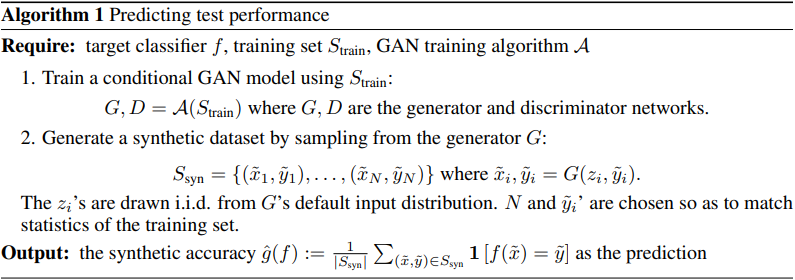Here, we use the pre-trained BigGAN+DiffAug from the StudioGAN library as the GAN, VGG-(11,13,19), ResNet-(18,34,50) and DenseNet-(121,169) as classifiers, and CIFAR-10 and Tiny The results of this attempt with ImageNet are shown in the following figure.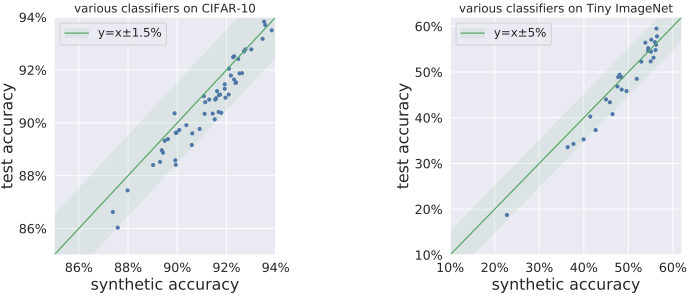As shown in the figure, for both datasets, the accuracy of the synthetic data consistently lies in the vicinity of the test accuracy for the various classifiers and serves as a good predictor.

### Evaluation in PGDL COMPETITION

Next, we make a similar attempt for the task in the NeurIPS 2020 Competition on Predicting the Generalization of Deep Learning.

However, since the goal of this task is to predict the Generalization Gap, not the test accuracy, Algorithm 1 has been modified accordingly. The rest of the experimental setup (e.g., GANs used) is the same as before. The results are as follows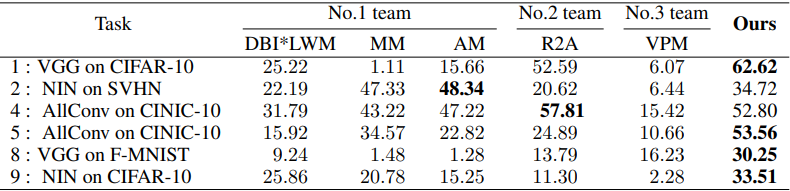Here the results are shown for the tasks except for the task where the GAN was not successfully trained.

In general, the proposed method significantly outperforms the top three teams. Note that hyperparameter search was not performed for all tasks, so there is a possibility that additional adjustments could improve the performance more.

### Evaluation in DEMOGEN BENCHMARK

Next, the results in the Deep Model Generalization benchmark (DEMOGEN) are as follows.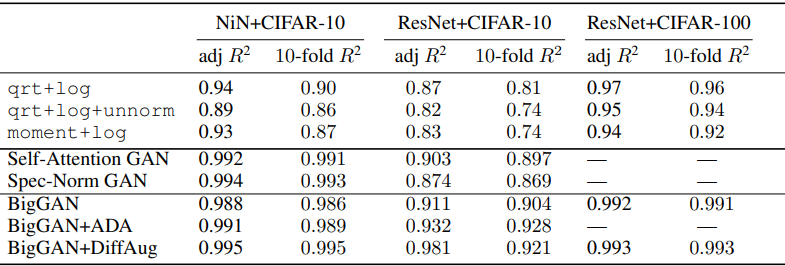In this table, the results are shown for the various GAN models used. Overall, the predictions made using GANs have shown very good results.

### The similarity between GAN samples and test set

To analyze why GAN samples show good properties for predicting test accuracy, we investigate the similarity between the synthetic data produced by GAN and the test set. For this purpose, we measure the similarity between the datasets by a metric named Class-conditional Frechet Distance.

Here, for a feature extractor $h$, the distance between two sets $S,\tilde{S}$ is given by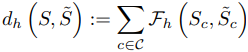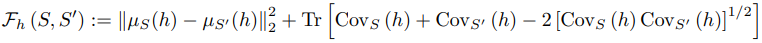The details of the definition are given in the original paper. The relationship between the inter-distributional distances for the synthetic dataset, training set, and test set, measured according to this metric, was as follows.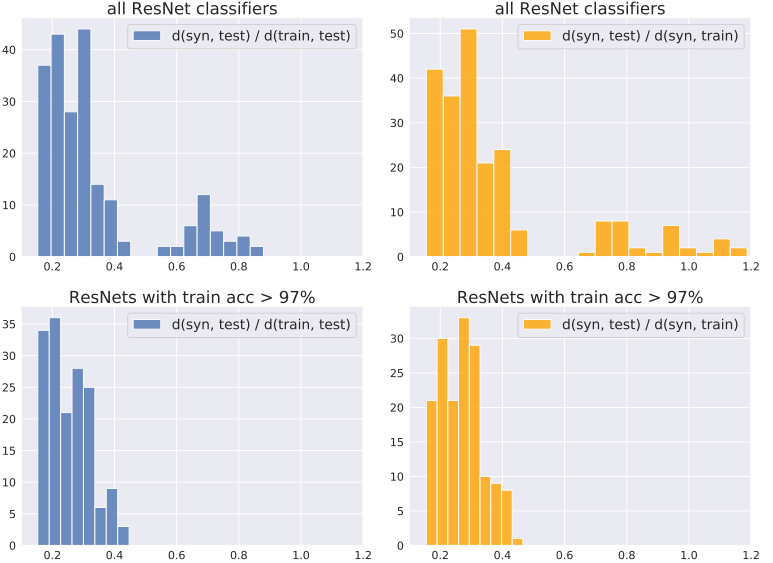Here, the upper part of the figure shows the ratio of the distance between distributions when a classifier with low accuracy is included, and the lower part shows the ratio of the distance between distributions when only a classifier with an accuracy of 97% or higher is used as a feature extractor. In general, $d_h(S_{syn}, S_{test}) < d_h(S_{syn}, S_{train})$ was observed, and this tendency was especially pronounced when a classifier with good performance was used.

This shows that the synthetic dataset is closer to the test set than to the training set. This result is quite surprising given that GANs are trained to increase the similarity between $S_{syn}$ and $S_{train}$.

To further validate this result, we calculated the distance between the distributions for the case where a different model from the GAN model Discriminator was used as a classifier in the same way.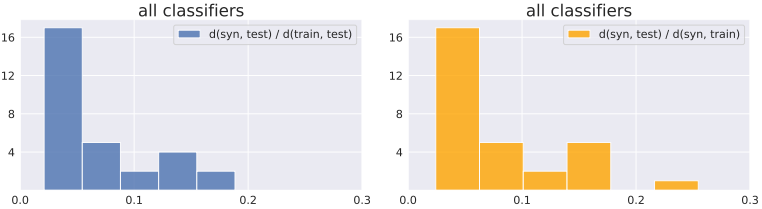In this case, the same results were obtained, again showing the phenomenon that the synthetic dataset is closer to the test set than the training set.

### The Effects of Data Augmentation

Finally, the plots of test and synthetic data accuracy with and without data augmentation when training the GAN and when training the classifier are shown below.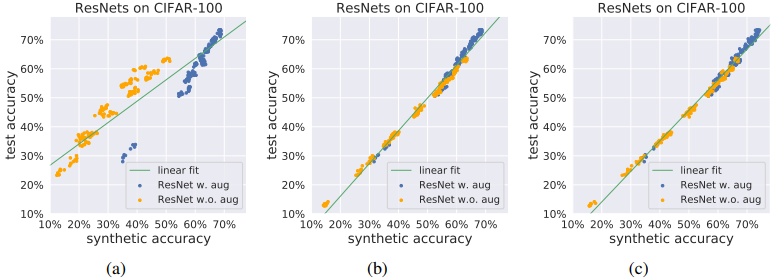Figure (a) shows the results of GANs trained by applying augmentation only to real image samples, (b) without augmentation, and (c) with differentiable augmentation. In addition, the dots are plotted in blue when there is data augmentation when training the classifier, and in orange when there is no augmentation. In general, we found that data augmentation is not always beneficial for generalization prediction.

The best results are likely to be obtained when differentiable augmentation is applied to both real and fake images and the Discriminator is regularized without manipulating the target distribution.

## summary

In this article, we have discussed studies that have addressed the novel idea of using synthetic data generated by GANs for generalized prediction and obtained useful results. In general, the results are surprising and counter-intuitive, with the distribution generated by GANs being closer to the test set than to the training set.

This is an interesting study that may provide an opportunity for further research, including a new theoretical understanding.

If you have any suggestions for improvement of the content of the article,